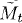# MARTINGALE REPRESENTATION THEOREM

The Itô integral defined in Chapter 32 is a martingale with respect to the underlying filtration. The martingale representation theorem states that the converse is also true. In this chapter, we introduce the martingale representation theorem.

# 36.1 Basic Concepts and Facts

Theorem 36.1 (Martingale Representation Theorem). Letbe the filtration generated by the Brownian motion, that is,be a square integrable martingale with respect to the filtration. Then Mt has a continuous versiongiven bywhere.

# 36.2 Problems

36.1. Let {Bt : t ≥ 0} be a Brownian motion with respect to a filtration. Let 0 ≤ a < b and X be a finitea-measurable random variable. ...

Get Measure, Probability, and Mathematical Finance: A Problem-Oriented Approach now with the O’Reilly learning platform.

O’Reilly members experience live online training, plus books, videos, and digital content from nearly 200 publishers.